## Free Statistics

of Irreproducible Research!

Author's title
Author*The author of this computation has been verified*
R Software Modulerwasp_edauni.wasp
Title produced by softwareUnivariate Explorative Data Analysis
Date of computationMon, 05 Nov 2012 07:01:55 -0500
Cite this page as followsStatistical Computations at FreeStatistics.org, Office for Research Development and Education, URL https://freestatistics.org/blog/index.php?v=date/2012/Nov/05/t1352116934th5b9mwkwi6khmy.htm/, Retrieved Sat, 23 Sep 2023 10:32:59 +0000
Statistical Computations at FreeStatistics.org, Office for Research Development and Education, URL https://freestatistics.org/blog/index.php?pk=185993, Retrieved Sat, 23 Sep 2023 10:32:59 +0000
QR Codes:Original text written by user:
IsPrivate?No (this computation is public)
User-defined keywords
Estimated Impact130
Family? (F = Feedback message, R = changed R code, M = changed R Module, P = changed Parameters, D = changed Data)
-     [Univariate Explorative Data Analysis] [time effect in su...] [2010-11-17 08:55:33] [b98453cac15ba1066b407e146608df68]
- R  D    [Univariate Explorative Data Analysis] [Sequency plot] [2012-11-05 12:01:55] [447cab31e466d1c88f957d20e303ed40] [Current]
- R         [Univariate Explorative Data Analysis] [Univariate EDA] [2012-12-16 15:34:24] [0dc867bfbaab36a894719867823e3cb9]
- R P         [Univariate Explorative Data Analysis] [Univariate EDA] [2012-12-19 13:07:25] [3ee3949b5f2daf713678f5a72e9e7041]
Feedback Forum

Post a new message
Dataseries X:
13
16
19
15
14
13
19
15
14
15
16
16
16
16
17
15
15
20
18
16
16
16
19
16
17
17
16
15
16
14
15
12
14
16
14
7
10
14
16
16
16
14
20
14
14
11
14
15
16
14
16
14
12
16
9
14
16
16
15
16
12
16
16
14
16
17
18
18
12
16
10
14
18
18
16
17
16
16
13
16
16
20
16
15
15
16
14
16
16
15
12
17
16
15
13
16
16
16
16
14
16
16
20
15
16
13
17
16
16
12
16
16
17
13
12
18
14
14
13
16
13
16
13
16
15
16
15
17
15
12
16
10
16
12
14
15
13
15
11
12
8
16
15
17
16
10
18
13
16
13
10
15
16
16
14
10
17
13
15
16
12
13

 Summary of computational transaction Raw Input view raw input (R code) Raw Output view raw output of R engine Computing time 5 seconds R Server 'Gwilym Jenkins' @ jenkins.wessa.net

\begin{tabular}{lllllllll}
\hline
Summary of computational transaction \tabularnewline
Raw Input & view raw input (R code)  \tabularnewline
Raw Output & view raw output of R engine  \tabularnewline
Computing time & 5 seconds \tabularnewline
R Server & 'Gwilym Jenkins' @ jenkins.wessa.net \tabularnewline
\hline
\end{tabular}
%Source: https://freestatistics.org/blog/index.php?pk=185993&T=0

[TABLE]
[ROW][C]Summary of computational transaction[/C][/ROW]
[ROW][C]Raw Input[/C][C]view raw input (R code) [/C][/ROW]
[ROW][C]Raw Output[/C][C]view raw output of R engine [/C][/ROW]
[ROW][C]Computing time[/C][C]5 seconds[/C][/ROW]
[ROW][C]R Server[/C][C]'Gwilym Jenkins' @ jenkins.wessa.net[/C][/ROW]
[/TABLE]
Source: https://freestatistics.org/blog/index.php?pk=185993&T=0

Globally Unique Identifier (entire table): ba.freestatistics.org/blog/index.php?pk=185993&T=0

As an alternative you can also use a QR Code:

The GUIDs for individual cells are displayed in the table below:

 Summary of computational transaction Raw Input view raw input (R code) Raw Output view raw output of R engine Computing time 5 seconds R Server 'Gwilym Jenkins' @ jenkins.wessa.net

 Descriptive Statistics # observations 162 minimum 7 Q1 14 median 16 mean 14.9506172839506 Q3 16 maximum 20

\begin{tabular}{lllllllll}
\hline
Descriptive Statistics \tabularnewline
# observations & 162 \tabularnewline
minimum & 7 \tabularnewline
Q1 & 14 \tabularnewline
median & 16 \tabularnewline
mean & 14.9506172839506 \tabularnewline
Q3 & 16 \tabularnewline
maximum & 20 \tabularnewline
\hline
\end{tabular}
%Source: https://freestatistics.org/blog/index.php?pk=185993&T=1

[TABLE]
[ROW][C]Descriptive Statistics[/C][/ROW]
[ROW][C]# observations[/C][C]162[/C][/ROW]
[ROW][C]minimum[/C][C]7[/C][/ROW]
[ROW][C]Q1[/C][C]14[/C][/ROW]
[ROW][C]median[/C][C]16[/C][/ROW]
[ROW][C]mean[/C][C]14.9506172839506[/C][/ROW]
[ROW][C]Q3[/C][C]16[/C][/ROW]
[ROW][C]maximum[/C][C]20[/C][/ROW]
[/TABLE]
Source: https://freestatistics.org/blog/index.php?pk=185993&T=1

Globally Unique Identifier (entire table): ba.freestatistics.org/blog/index.php?pk=185993&T=1

As an alternative you can also use a QR Code:

The GUIDs for individual cells are displayed in the table below:

 Descriptive Statistics # observations 162 minimum 7 Q1 14 median 16 mean 14.9506172839506 Q3 16 maximum 20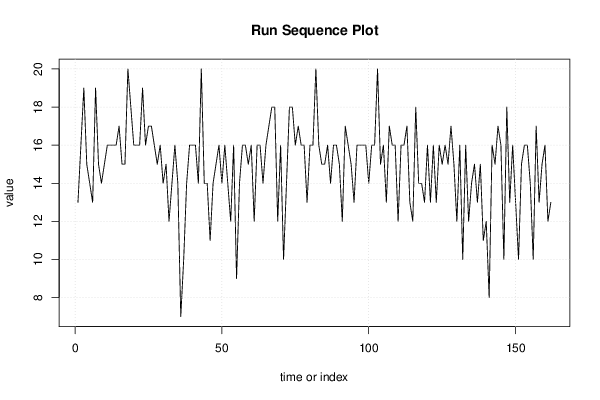PNG link Postscript link PDF link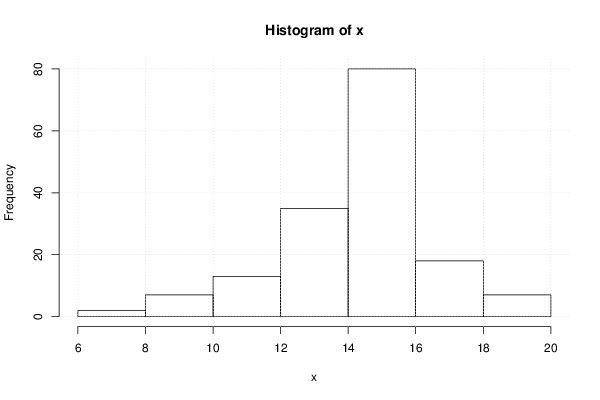PNG link Postscript link PDF link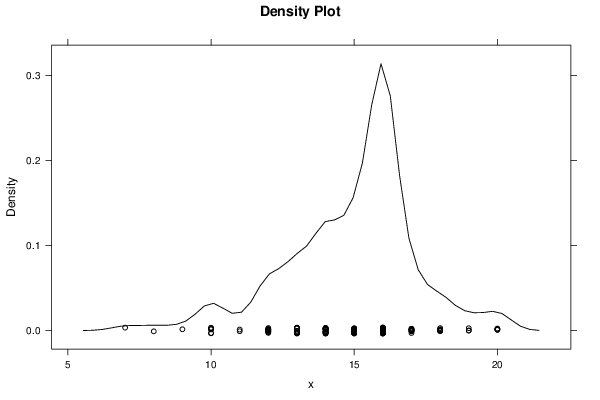PNG link Postscript link PDF link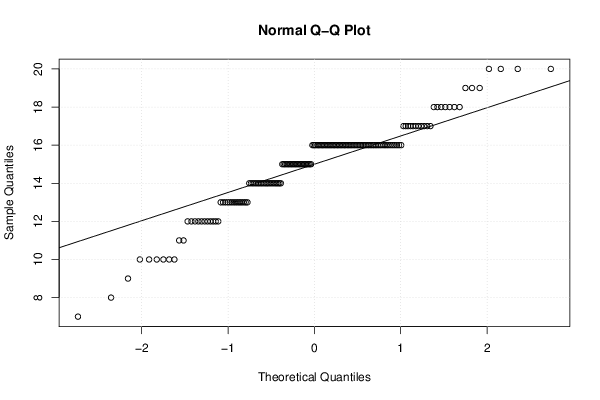PNG link Postscript link PDF link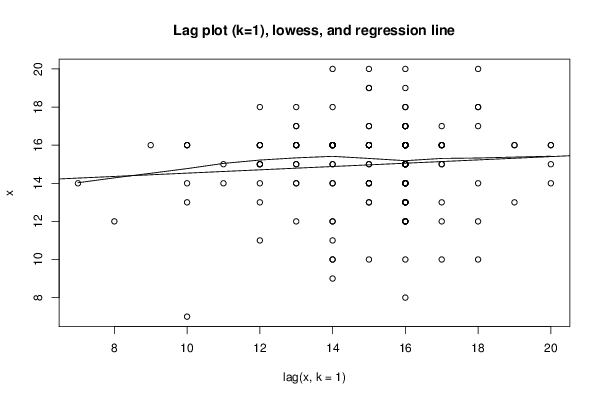PNG link Postscript link PDF link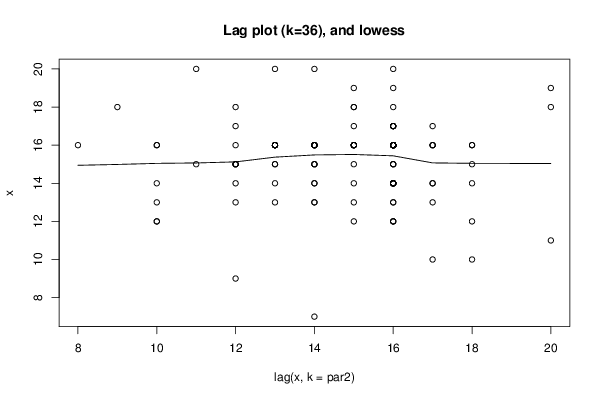PNG link Postscript link PDF link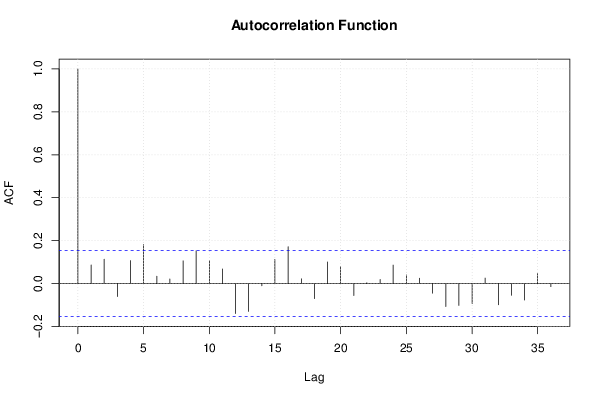PNG link Postscript link PDF link

Parameters (Session):
par1 = 0 ; par2 = 36 ;
Parameters (R input):
par1 = 0 ; par2 = 36 ;
R code (references can be found in the software module):
par1 <- as.numeric(par1)par2 <- as.numeric(par2)x <- as.ts(x)library(lattice)bitmap(file='pic1.png')plot(x,type='l',main='Run Sequence Plot',xlab='time or index',ylab='value')grid()dev.off()bitmap(file='pic2.png')hist(x)grid()dev.off()bitmap(file='pic3.png')if (par1 > 0){densityplot(~x,col='black',main=paste('Density Plot   bw = ',par1),bw=par1)} else {densityplot(~x,col='black',main='Density Plot')}dev.off()bitmap(file='pic4.png')qqnorm(x)qqline(x)grid()dev.off()if (par2 > 0){bitmap(file='lagplot1.png')dum <- cbind(lag(x,k=1),x)dumdum1 <- dum[2:length(x),]dum1z <- as.data.frame(dum1)zplot(z,main='Lag plot (k=1), lowess, and regression line')lines(lowess(z))abline(lm(z))dev.off()if (par2 > 1) {bitmap(file='lagplotpar2.png')dum <- cbind(lag(x,k=par2),x)dumdum1 <- dum[(par2+1):length(x),]dum1z <- as.data.frame(dum1)zmylagtitle <- 'Lag plot (k='mylagtitle <- paste(mylagtitle,par2,sep='')mylagtitle <- paste(mylagtitle,'), and lowess',sep='')plot(z,main=mylagtitle)lines(lowess(z))dev.off()}bitmap(file='pic5.png')acf(x,lag.max=par2,main='Autocorrelation Function')grid()dev.off()}summary(x)load(file='createtable')a<-table.start()a<-table.row.start(a)a<-table.element(a,'Descriptive Statistics',2,TRUE)a<-table.row.end(a)a<-table.row.start(a)a<-table.element(a,'# observations',header=TRUE)a<-table.element(a,length(x))a<-table.row.end(a)a<-table.row.start(a)a<-table.element(a,'minimum',header=TRUE)a<-table.element(a,min(x))a<-table.row.end(a)a<-table.row.start(a)a<-table.element(a,'Q1',header=TRUE)a<-table.element(a,quantile(x,0.25))a<-table.row.end(a)a<-table.row.start(a)a<-table.element(a,'median',header=TRUE)a<-table.element(a,median(x))a<-table.row.end(a)a<-table.row.start(a)a<-table.element(a,'mean',header=TRUE)a<-table.element(a,mean(x))a<-table.row.end(a)a<-table.row.start(a)a<-table.element(a,'Q3',header=TRUE)a<-table.element(a,quantile(x,0.75))a<-table.row.end(a)a<-table.row.start(a)a<-table.element(a,'maximum',header=TRUE)a<-table.element(a,max(x))a<-table.row.end(a)a<-table.end(a)table.save(a,file='mytable.tab')